# Computing Sections of a Graph Which Can Be Removed By Removing Two Edges

• MATLAB
person123
TL;DR Summary
I would like to write an algorithm to do this in MATLAB to analyze a network of atomic bonds.
Hi. I have graph which I am analyzing in MATLAB. I would like to determine sections of the graph which could be removed from the rest of the graph by removing only 2 edges. (The graph represents the network of atomic bonds from a simulation of silica, and I am attempting to locate strands of atoms). Is there an efficient algorithm for computing this? Here is a sketch of what I mean: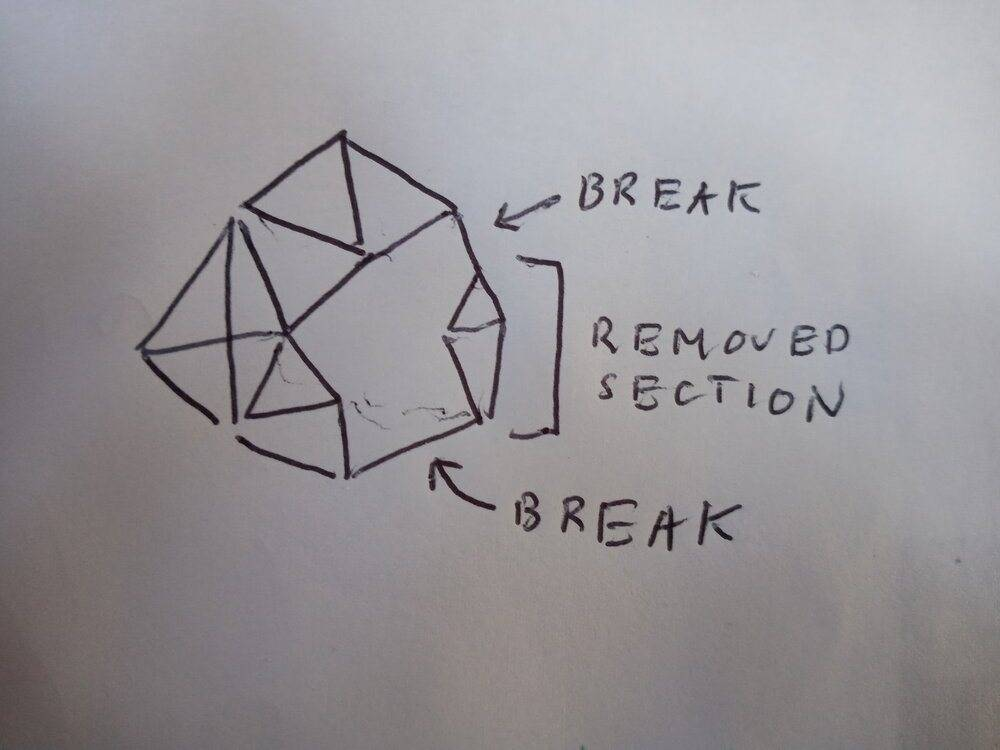Thank you!

Mentor

Convert edges to vertices and vertices to edges (connecting all associated new vertices with each other) and test if the new graph is 3-vertex-connected:

https://mathworld.wolfram.com/BiconnectedGraph.html
https://reference.wolfram.com/language/ref/KVertexConnectedGraphQ.html

No idea about the runtime of that algorithm, and no idea if Matlab has one on its own.

That's only telling you if such a structure exists, but presumably somewhere in the code it also identifies these structures.

Looking for "2-edge connected" as keyword might show something as well.

Last edited:
person123
The graph has (approximately) 2500 vertices and 6500 edges. The graph changes over time, so it should be done iteratively for each step (125 in my case). I plan on having larger graphs in the future however, maybe on the order of 10s or even 100s of thousands of vertices. (I may have to use my university's computing cluster for this).

I can't find any tools in MATLAB for either switching vertices and edges or determining k-vertex-connectedness though.

I think an easier alternative might be to determine sections which can be removed from the graph by removing two vertices which each only have two edges. This is of course a different problem, but I think if I had strands of atoms, there would be atoms on either side of the strand only bonded to two other atoms. Here's a sketch: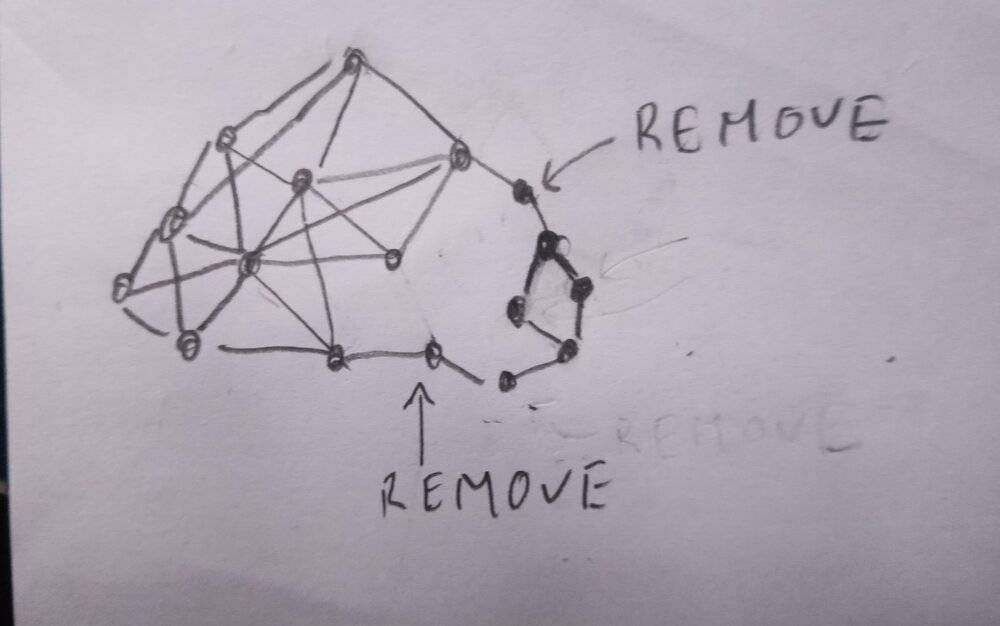For this problem, I think I could iterate through all pairs of vertices which have two edges (there shouldn't be many vertices with only two edges) and see if their removal leads to separate graphs.

The smaller subgraph (the one which is the strand of atoms) would like have multiple vertices with only two edges (it might actually be the only place where there's vertices with two edges). Because of that, I think I could remove all vertices with two edges which are within the smaller subgraph from the iteration process to improve efficiency. (They would just find strands which are part of a larger strand). Here is a sketch: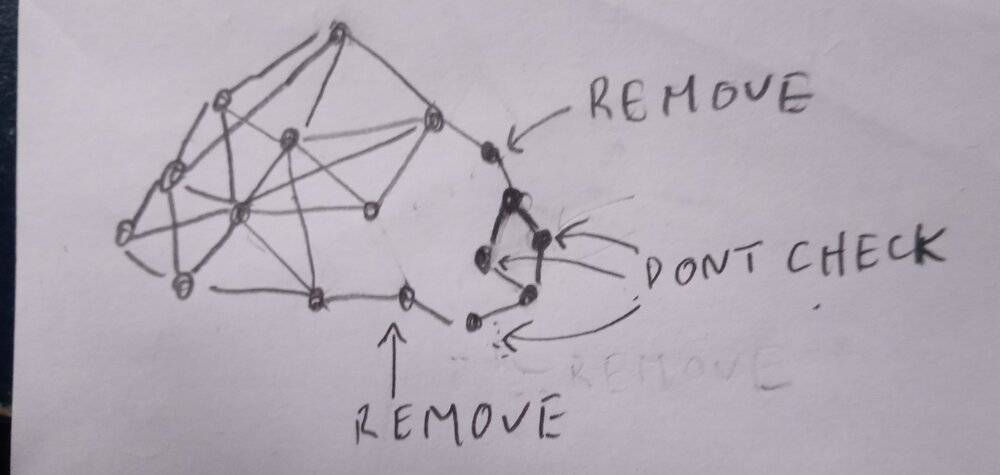I think this is something I could code easily (removing vertices and finding separate graphs is built into MATLAB).

Does the approach seem reasonable?

Mentor
Chains of atoms certainly help. Start with atoms with only two connections, extend them to mark the full chain (this is trivial as you can just keep following the connections), then check pairs of chains.

It should also be possible to make a meta-graph out of densely connected regions as vertices with these chains as edges between them.

person123
extend them to mark the full chain (this is trivial as you can just keep following the connections)
I'm a bit confused about this step. How would it know when to stop? I'm not assuming these are chains of single atoms. It could be a cluster of atoms like shown: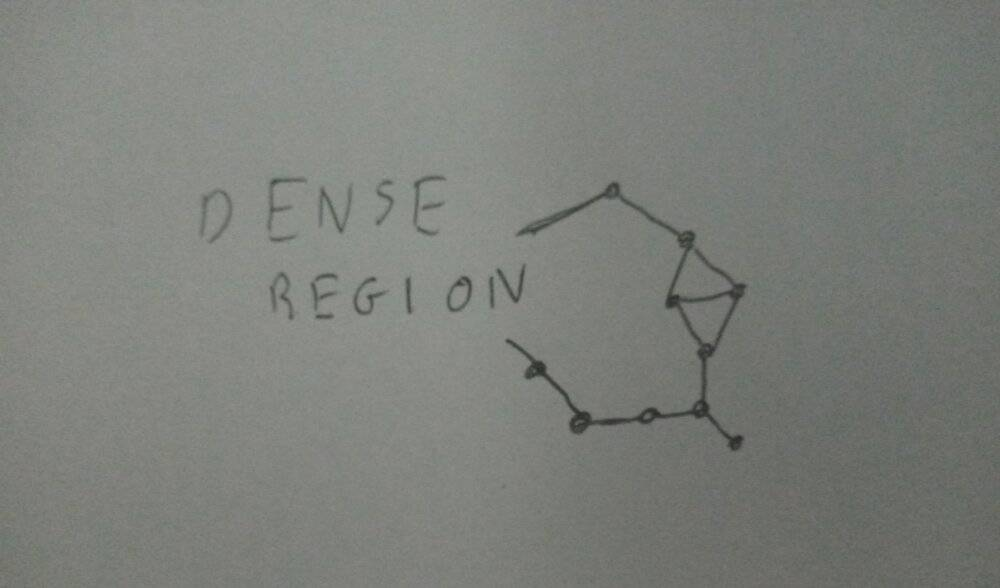My idea was to say the chain is bounded by atoms with only to bonds, but there could be atoms with more than two bonds within the chain.

(I imagine this would be easier if I showed pictures of the simulations, but it's unpublished research and I'm not sure what the rules are on what I can show).

Mentor
Smaller clusters are still reasonably easy to identify I think. Make a list of connected atoms, check all their connections recursively. You either run into a dead end before you reached N atoms (end of the chain), you reach a single open connection (cluster) or you reach N atoms (bulk). If your whole chain can look like a ladder things get more complicated.

•person123
person123
I found that it's okay to show it, so this is what one of them looks like: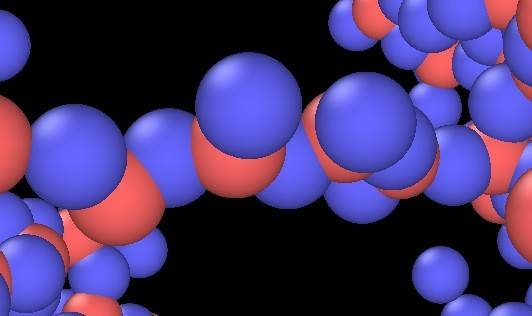Is N the maximum number of atoms in the chain?

Mentor
The N in my post would be the maximum number of atoms in small clusters as part of a larger chain. If you find more atoms that are densely connected then you are not in a chain any more.